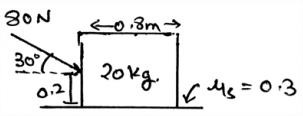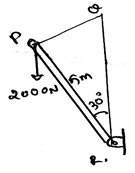Courses

# Test: Rolling Resistance

## 15 Questions MCQ Test Engineering Mechanics | Test: Rolling Resistance

Description
This mock test of Test: Rolling Resistance for Mechanical Engineering helps you for every Mechanical Engineering entrance exam. This contains 15 Multiple Choice Questions for Mechanical Engineering Test: Rolling Resistance (mcq) to study with solutions a complete question bank. The solved questions answers in this Test: Rolling Resistance quiz give you a good mix of easy questions and tough questions. Mechanical Engineering students definitely take this Test: Rolling Resistance exercise for a better result in the exam. You can find other Test: Rolling Resistance extra questions, long questions & short questions for Mechanical Engineering on EduRev as well by searching above.
QUESTION: 1

### What does the moment of the force measure in the rolling of the body?

Solution:

The moment of the force measures the tendency of the rotation of the body along any axis, whether it be the centroid axis of the body, or any of the outside axis. The couple moment is produced by two forces, not by a single force. The total work done is the dot product of force and distance not the cross.

QUESTION: 2

### Determine the vertical force acting in the given figure.Solution:

The net forces acting on the body is shown by the help of the resultant forces. There are two types, first the frictional and the second is the normal. This is because the resultant forces have the sum of all the forces which are acting on the direction which is same.

QUESTION: 3

### If a car is moving forward, what is the direction of the moment of the moment caused by the rolling of the tires, assume non slippery surface?

Solution:

When you curl your wrist in the direction in which the tires are moving then you will find that the thumb is pointing outwards. That is outwards the body of the car. This phenomena is also observed in rainy seasons. When cars travel on the roads, the water is thrown outside from the tires, due to moment.

QUESTION: 4

The moment of the force is the product of the force and the perpendicular distance of the axis and the point of action of the force. Is this also true for rolling?

Solution:

The moment is the product of the force applied to the body and the perpendicular distance of the point of action of the force to the axis about which the body is being rotated. That is the moment is the cross product of the force and the distance between the axis and the point of action. Yes this is also true for rolling.

QUESTION: 5

For the rolling of the body the calculation of the moment of the force about the axis of rolling, the cross product table, i.e. the 3X3 matrix which is made for doing the cross product having 3 rows, contains three elements. Which are they from top to bottom?

Solution:

The 3X3 matrix which is being made is having axis coordinates, point coordinates and the force coordinates. They are from top to bottom placed. The order cannot be changed. Or if changed then one needs to apply the negative sign appropriately. Negative because the directions gets reversed.

QUESTION: 6

Find the moment of the force about the point R.Solution:

As we know that the moment is the cross product of the distance and the force we will try to apply the same here. We see that the perpendicular distance is 5m. Thus we get the distance. And hence multiply it with the force, 5x2000xsin30. Because the force component perpendicular to the distance need to be taken.

QUESTION: 7

In rolling there is the involvement of the vector math. So for rolling which of the following is correct? (For A representing the vector representation of the axis of rotation, r the radius vector and F the force vector)

Solution:

The correct form of the equation is given by A.(rxF). Where A represents the vector representation of the axis of rotation, r the radius vector and F the force vector. This is usually done for determining the moment of the force about the axis. That is if body is being rotated by the force about an axis.

QUESTION: 8

In the equation A.(rxF), the r vector is what?

Solution:

The r in the equation A.(rxF) is the radius vector of the rolling body. That is it is the vector which is having the start point at the axis of rolling and the end point at the point of action of the force on the rolling body. This vector is being crossed by the force vector, which is then followed by the dot product with the axis vector.

QUESTION: 9

The ___________ forces do not cause the rotation/rolling of the body if the rotation is considered in about the axis of the body or the centroid axis of the body.

Solution:

The concurrent forces are the which are somewhere touching the axis of rotation. If any of the force is touching that axis, that force is not considered, or is insufficient to cause a rotation. If a force is concurrent then the perpendicular distance of the force from the line of axis is zero, thus no rotation. As we know rotation is caused by moment.

QUESTION: 10

For the rolling of the body right handed coordinate system means (consider the mentioned axis to be positive)?

Solution:

As right handed coordinate system means that you are curling your fingers from positive x-axis towards y-axis and the thumb which is projected is pointed to the positive z-axis. Thus visualizing the same and knowing the basic members of axis will not create much problem. The right handed coordinate system is universal throughout.

QUESTION: 11

In the equation Wa/r generally used in the rolling frictional calculations, what does each stands for?

Solution:

The rolling of the cylinder is termed as perfect rolling only when the cylinder is rolling at a constant velocity. Also the rolling should be along the rigid surface. The normal forces are acting perpendicular to the surface of the rolling. And for rolling equation Wa/r is generally used.

QUESTION: 12

Which statement is correct about the vector F acting parallel to the direction of the motion of the rolling body?

Solution:

As we know the α, β and γ are the angles made by the x, y and z-axis respectively. Thus, is the magnitude of the vector is F, the F= Fcos β + Fcos α + Fcosγ. Which means the force is the resultant of all its axis’ components. The force driving the rolling motion of the body can be divided like this.

QUESTION: 13

What if the moment of the force calculated about the axis of rolling is negative?

Solution:

It means that the direction of the rolling is in the opposite sense as imagined. We can’t say about the direction of the force or the direction of the radius vector. But yes we can say about the direction of the rolling as it is the thing which is going to be calculated. Rest all the parts are fixed. They can’t be altered.

QUESTION: 14

In the equation A.(rxF) the r is heading from ______________ and ending at _____________

Solution:

It is the radius vector. The radius vector is always from the axis of rolling to the point of action of the force on the body. Which means that the radius vector is not on any point on the force vector. Rather it ending at the point on the force vector, where it is being in contact of the body.

QUESTION: 15

Rotation is termed as rolling when _______

Solution:

The rolling of the cylinder is termed as perfect rolling only when the cylinder is rolling at a constant velocity. Also the rolling should be along the rigid surface. The normal forces are acting perpendicular to the surface of the rolling.